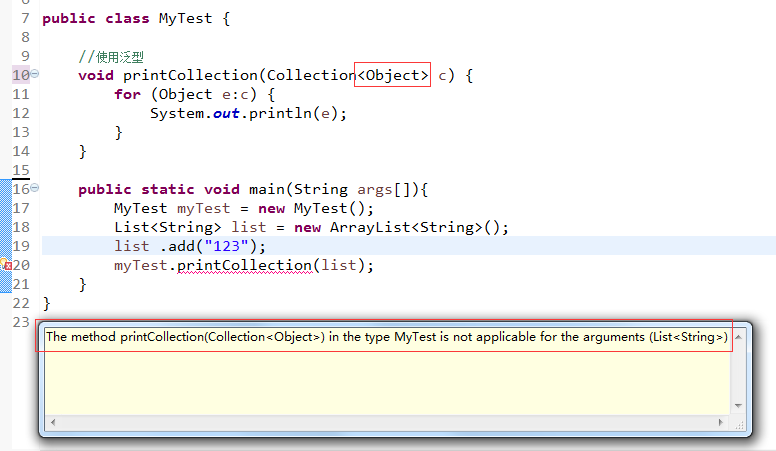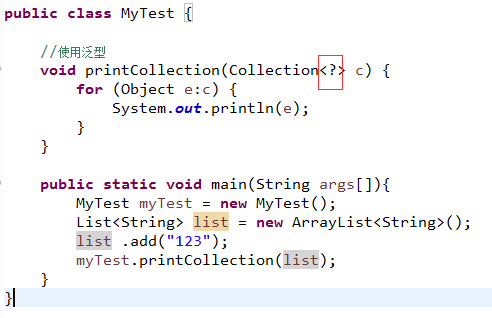# Java基础之泛型

## 一、泛型的理解与简单使用

#### 1.1、泛型在接口上的使用：

package com.luo.test;

public interface TestInterface<T> {

String objectToString(T o);

}

package com.luo.test;

public class TestInterfaceImpl<T> implements TestInterface<T> {

public String objectToString(T o) {
return o.toString();
}

public static void main(String args[]){
TestInterfaceImpl<Integer> testInterfaceImpl = new TestInterfaceImpl<Integer>();
Integer integer = new Integer(123);
System.out.println(testInterfaceImpl.objectToString(integer));
}

}

#### 1.2、泛型在类上单独使用（不实现接口）：

package com.luo.test;

public class ClassTest<T> {

private T ob; // 定义泛型成员变量

public T getOb() {
return ob;
}

public void setOb(T ob) {
this.ob = ob;
}

public void showObType() {
System.out.println("T的实际类型是: " + ob.getClass().getName());
}

public static void main(String args[]){
ClassTest<Integer> classTest = new ClassTest<Integer>();
classTest.setOb(123);
classTest.showObType();
}

}

#### 1.3、泛型在方法上单独使用：

package com.luo.test;

import java.util.ArrayList;
import java.util.List;

public class MyTest {

public <T> List<T> write(T[] array){
List<T> list = new ArrayList<T>();
for (int i = 0; i < array.length; i++) {
}
return list;
}

public static void main(String args[]){

}
}

## 二、泛型的高级使用

#### 2.1、通配符“？”Collection<Object>并不是所有集合的超类。
List<Object>, List<String>是两种不同的类型，他们之间没有继承关系，即使String继承了Object。#### 2.2、边界通配符“？extends”

public abstract class Shape {
public abstract void draw(Canvas c);
}

public class Circle extends Shape {
public void draw(Canvas c) { ... }
}

public class Rectangle extends Shape
private int x,y,width,height;
public void draw(Canvas c) { ... }
}
为了画出集合中所有的形状，我们可以定义一个函数，该函数接受带有泛型的集合类对象作为参数。但是不幸的是，我们只能接收元素类型为Shape的List对象，而不能接收类型为List<Cycle>的对象，这在前面已经说过。为了解决这个问题，所以有了边界通配符的概念。这里可以采用public void drawAll(List<? extends Shape> shapes)来满足条件，这样就可以接收元素类型为Shape子类型的列表作为参数了。
//使用边界通配符的版本
public void drawAll(List<？exends Shape> shapes) {
for (Shapes:shapes) {
s.draw(this);
}
}

#### 2.3、通配符“？super”

List<Shape> shapes = new ArrayList<Shape>();
List<? super Cicle> cicleSupers = shapes;
cicleSupers.add(new Cicle()); //OK, subclass of Cicle also OK
cicleSupers.add(new Shape()); //ERROR

## 三、总结

#### 3.1、 类型擦除概念

/*会导致编译时错误*/
public class Erasure{
public void test(List<String> ls){
System.out.println("Sting");
}
public void test(List<Integer> li){
System.out.println("Integer");
}
}

#### 3.2、方法重载

/*代码一：编译时错误*/
public class Erasure{
public void test(int i){
System.out.println("Sting");
}
public int test(int i){
System.out.println("Integer");
}
}
/*代码二：正确 */
public class Erasure{
public void test(List<String> ls){
System.out.println("Sting");
}
public int test(List<Integer> li){
System.out.println("Integer");
}
}

#### 3.3、泛型类型是被所有调用共享的

List<String>l1 = new ArrayList<String>();
List<Integer>l2 = new ArrayList<Integer>();
System.out.println(l1.getClass() == l2.getClass()); //True

#### 3.4、instanceof

Collection cs = new ArrayList<String>();
if (cs instanceof Collection<String>){…}// compile error.如果改成instanceof Collection<?>则不会出错。

#### 3.5、泛型数组问题

List<String>[] lsa = new ArrayList<String>; //compile error.

List<String>[] lsa = new ArrayList<String>; // 实际上并不允许这样创建数组
Object o = lsa;
Object[] oa = (Object[]) o;
List<Integer>li = new ArrayList<Integer>();
oa = li;// unsound, but passes run time store check
String s = lsa.get(0); //run-time error - ClassCastException
因此只能创建带通配符的泛型数组，如下面例子所示，这回可以通过编译，但是在倒数第二行代码中必须显式的转型才行，即便如此，最后还是会抛出类型转换异常，因为存储在lsa中的是List<Integer>类型的对象，而不是List<String>类型。最后一行代码是正确的，类型匹配，不会抛出异常。
List<?>[] lsa = new List<?>; // ok, array of unbounded wildcard type
Object o = lsa;
Object[] oa = (Object[]) o;
List<Integer>li = new ArrayList<Integer>();
Integer it = (Integer)lsa.get(0); // OK&nbsp;